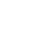### C++ Factory Method Pattern Example

In this article, we will learn how to use and implement the Factory method pattern in C++ with an example.

# The factory design pattern overview

• Factory Pattern is one of the Creational Design Pattern.
• In the Factory pattern, we create an object without exposing the creation logic to the client and refer to newly created objects using a common interface.
• The factory design pattern is used when we have a superclass with multiple sub-classes and based on input, we need to return one of the sub-class. This pattern takes out the responsibility of the instantiation of a class from the client program to the factory class.

# Factory Method Example

In this example, we're going to create a Shape interface and concrete classes implementing the Shape interface.

```#include <iostream>
#include <string>

using namespace std;

/*
* Define an interface for creating an object, but let
* subclasses decide which class to instantiate.
*/

class Shape {
public:
virtual void Draw() = 0;
static Shape* ShapeFactory(string type);
};

class Circle : public Shape {
public:
void Draw() { cout << "I am circle" << endl; }
friend class Shape;
};

class Square : public Shape {
public:
void Draw() { cout << "I am square" << endl; }
friend class Shape;
};

class Rectangle : public Shape {
public:
void Draw() { cout << "I am rectangle " << endl; }
friend class Shape;
};

Shape* Shape::ShapeFactory(string type) {
if ( type == "circle" ) return new Circle();
if ( type == "square" ) return new Square();
if ( type == "rectangle" ) return new Rectangle();
return NULL;
}

int main(){
Shape* obj1 = Shape::ShapeFactory("circle");
Shape* obj2 = Shape::ShapeFactory("square");
Shape* obj3 = Shape::ShapeFactory("rectangle");
obj1->Draw();
obj2->Draw();
obj3->Draw();
}```

# Output

``````I am circle
I am square
I am rectangle``````

# When to use

• a class can't anticipate the class of objects it must create
• a class wants its subclasses to specify the objects it creates
• classes delegate responsibility to one of several helper subclasses, and you want to localize the knowledge of which helper subclass is the delegate

# Benefits of Factory Pattern

• Factory design pattern provides an approach to code for interface rather than implementation.
• Factory pattern removes the instantiation of actual implementation classes from client code. Factory pattern makes our code more robust, less coupled, and easy to extend. For example, we can easily change PC class implementation because the client program is unaware of this.
• Factory pattern provides abstraction between implementation and client classes through inheritance.

# Related C++ Design Patterns

1.